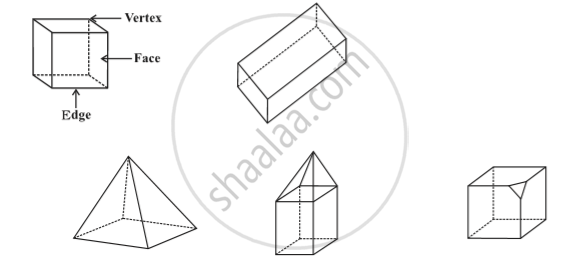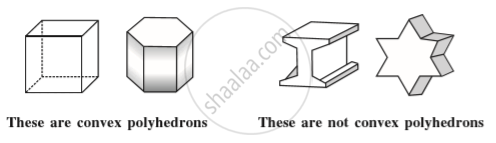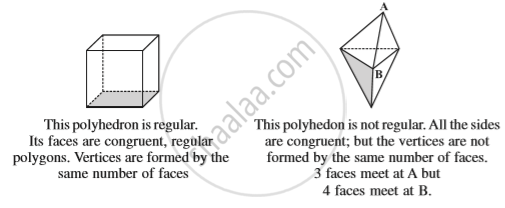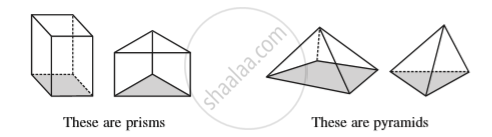Share

# Faces, Edges and Vertices

#### notes

Look at the following solids .Each of these solids is made up of polygonal regions which are called its faces; these faces meet at edges which are line segments; and the edges meet at vertices which are points. Such solids are called polyhedrons.

Convex polyhedrons: You will recall the concept of convex polygons. The idea of convex polyhedron is similar.Regular polyhedrons: A polyhedron is said to be regular if its faces are made up of regular polygons and the same number of faces meet at each vertex.Two important members of polyhedron family around are prisms and pyramids.A prism is a polyhedron whose base and top are congruent  polygons and whose other faces, i.e., lateral faces are parallelograms in shape.
On the other hand, a pyramid is a polyhedron whose base is a polygon (of any number of sides) and whose lateral faces are triangles with a common vertex. (If you join all the corners of a polygon to a point not in its plane, you get a model for pyramid).

For any polyhedron, F + V – E = 2
where ‘F’ stands for number of faces, V stands for number of vertices and E stands for number of edges. This relationship is called Euler’s formula.

### Shaalaa.com

Euler's Formula [00:04:28]
S
0%

S